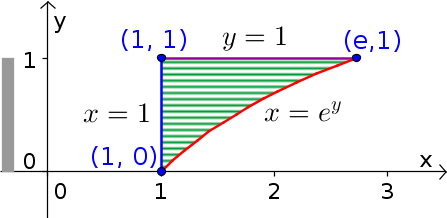# Math Insight

### Image: Change order of integration example region with exponential, x firstExample region illustrating process of changing the order of integration in double integrals, shown with x as the inner integral. For a given value of $y$, $x$ ranges from the line $x=1$ (blue) to the curve $x=e^y$ (red), as illustrated by the horizontal hashing over the region. The total range of $y$ over the region is from zero to one, as illustrated by the gray bar at left.

Source image file: double_integral_change_order_example_exp_x.ggb
Source image type: Geogebra